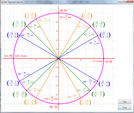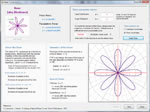"Trigonometry is a sine of the times."
- Author Unknown

## Covered Topics

Currently, the Trigonometry app is not as far along as the other three apps and contains only the following capabilities at this time: definitions and values of trigonometric functions, graphs of trigonometric functions, the Unit Circle and Polar Coordinates. Upon completion, the app will also cover SOH-CAH-TOA, inverse trigonometric functions, oblique triangles, functions of two angles, trigonometric identities, trigonometric equations and vectors.

The app also contains a glossary of terms relating to Geometry and Trigonometry.